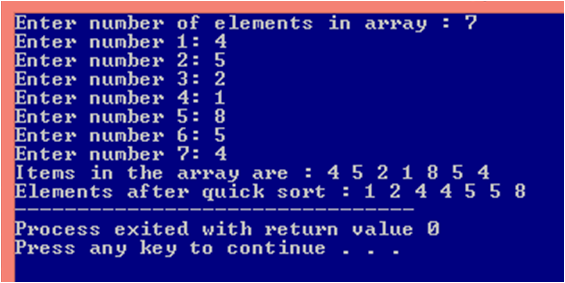# Quick Sort Program In C

## QUICK SORT IN C

Back to Quick Sort

Quick Sort is another sorting algorithm that is based on divide and conquer approach. Unlike merge sort that divides input elements according to their position in array, quick sort divides input element according to their values. Here is a three-step divide and conquer process for sorting a typical sub-array.

#### Combine :Because the sub-arrays are already sorted, no work is needed to combine them : the entire array a[ p . . r ] is now sorted.

Lets understand Quick Sort with the help of an example :

Array Elements ( List ) : 2 8 7 1 3 5 6 4

Steps :

• Select the pivot element ( 4 in this case )
• Rearrange elements such that elements before pivot element are less than it and element after pivot element are greater than it. This arrangement of elements in accordance with the pivot element is called partition operation.
• Sort the sub-array recursively.Steps for Quick Sort
• The above image shows the partition of Array elements ( Initial list ).
• Similarly the two sub-array before and after pivot element are partitioned.
• The complete steps of quick sort is shown below.

## QUICK SORT ALGORITHM

`// Algorithm for Quick Sort`
```
```
```QUICKSORT(a, p, r)
if p<r
q = PARTITION(a, p, r)
QUICKSORT(a, p, q-1)```
`    QUICKSORT(a, q+1, r)`

Partitioning the array :

```PARTITION(a, p, r)
x=a[r]
i=p-1
```
```  for j=p to r-1
if(a[j]<=x)
i=i+1
swap a[i] with a[j]```
`  end loop`
```
swap a[i+1] with a[r]```
`  return i+1`

## QUICK SORT PROGRAM IN C

```//Quick sort program ( code ) in c

#include<stdio.h>

void quicksort(int a[],int p, int r);
int partition(int a[],int p, int r);

int main()
{
int a,i,n;
printf("Enter number of elements in array : ");
scanf("%d",&n);

// Inputting elements
for(i=0;i<n;i++)
{
printf("Enter number %d: ",i+1);
scanf("%d",&a[i]);
}

// Displaying Elements before quick sort
printf("Items in the array are : ");
for(i=0;i<n;i++)
{
printf("%d ",a[i]);
}

//Applying quick sort
quicksort(a,0,n-1);

// Displaying elements after quick sort
printf("\nElements after quick sort : ");
for(i=0;i<n;i++)
{
printf("%d ",a[i]);
}
return 0;
}

void quicksort(int a[],int p, int r)
{
int q;
if(p<r)
{```
```     // q - index at which pivot element lies
q=partition(a,p,r);```
```
```
```     // Sorting sub-array recursively
quicksort(a,p,q-1);
quicksort(a,q+1,r);
}
}

int partition(int a[],int p, int r)
{
int temp;```
`   int i=p-1;`
`   int j;`
```
```
```   // pivot element around which partition is done
int x=a[r];```
```
for(j=p;j<=r-1;j++)
{
if(a[j]<=x)
{
i=i+1;
temp=a[i];
a[i]=a[j];
a[j]=temp;
}
}

temp=a[i+1];
a[i+1]=a[r];
a[r]=temp;
return (i+1);
}
```

## Quick Sort Program OutputQuick Sort : Quick Sort Program Output

#### Related Posts

Previous
Next Post »